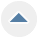Basic Crochet Abbreviations Chart

Category Crochet
When working on a crochet pattern you will need to know a variety of crochet stitch abbreviations to follow the instructions. This is a page about basic crochet abbreviations chart.

Related Product:

Tip: Basic Crochet Abbreviations Chart

June 27, 2007

Here are some basic crochet abbreviations:

alt = alternate
approx = approximately
beg = begin(ning)
bet = between
BL/bk lp = back loop(s)
BLO = back loop only
bo = bobble
BP = back post
BPdc = back post double crochet(s)
BPsc = back post single crochet(s)
BPtr = back post triple crochet(s)
CA = color A
CB = color B
CC = contrasting color
ch(s) = chain(s)
ch- = refers to chain previously made/worked
ch-sp = chain space
CL(s) =cluster(s)
cm = centimeter
cont = continue
dc = double crochet
dc2tog = double crochet 2 stitches together
dec = decrease
dtr/dtrc = double triple/treble crochet
ea = each
est = established
FLO = front loop only
foll = follow
FP = front post
FPdc = front post double crochet(s)
FPsc = front post single crochet(s)
FPtr/FPtrc = front post triple/treble crochet(s)
FL/ft lp = front loop(s)
g = gram
grp(s) = groups
hdc = half double crochet(s)
hk = hook
inc = increase
lp(s) = loop(s)
lp st = loop stitch
MC = main color
m = meters
mm = millimeters
oz = ounce(s)
pat(s) = pattern(s)
PC(s) = popcorn(s)
pm = placemaker
prev = previous
rem = remain
rep = repeat(s)
rev sc/reverse sc = reverse single crochet
rnd(s) = round(s)
RS = right side

sc = single crochet
sc2tog = single crochet 2 stitches together
sk = skip(ped)
sl st = slip stitch
sp(s) = space(s)
st(s) = stitch(es)
tch/t-ch = turning chain
tog = together
tr/trc = treble/triple crochet
tr tr/trtrc =triple treble crochet/triple triple crochet(s)
WS = wrong side
X-st = cross-stitch
yd = yard(s)
yo = yarn over
yoh = yarn over hook